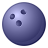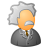# Calculate Value gives 1, not string

1743
2
10-08-2012 07:26 AMNew Contributor
My model is to upload an xy table, define, and project it, while using the worksheet name as the final name of the projected data. This works great. When I add a clip function, so points are clipped to a study area, the \$ that arc adds to the table name is an invalid character.

Using calculate value to strip the \$, I get a 1, not the string minus the \$. So output file is "1_clip.shp", rather than the "wksheet name_clip.shp". When Calc Value is in model, the output is listed as a greyed out 1. Seems odd, like the expression isn't working? I've used several expressions and code blocks with the same results. The upload, project and clip works smoothly, just gives it the wrong file name. Any ideas? (In case you can't tell, I'm not a strong python person, yet)

# Import arcpy module
import arcpy

arcpy.ImportToolbox("Model Functions")

# Set Geoprocessing environments

# Script arguments
XY_Table = arcpy.GetParameterAsText(0)

Define_Coordinate_System = arcpy.GetParameterAsText(1)

Projection = arcpy.GetParameterAsText(2)

Geographic_Transformation = arcpy.GetParameterAsText(3)

Study_Area = arcpy.GetParameterAsText(4)

Projected_and_Clipped_Data = arcpy.GetParameterAsText(5)
if Projected_and_Clipped_Data == '#' or not Projected_and_Clipped_Data:
Projected_and_Clipped_Data = "X:\\Tools\\XY\\point_upload\\Data\\%fixed%_Clip.shp" # provide a default value if unspecified

# Local variables:
xy_event = XY_Table
v_defined = xy_event
defined_data = v_defined
v_projected = defined_data
Value = XY_Table

# Process: Parse Path
arcpy.ParsePath_mb(XY_Table, "NAME")

# Process: Make XY Event Layer
tempEnvironment0 = gp.scratchWorkspace
tempEnvironment1 = gp.workspace
arcpy.MakeXYEventLayer_management(XY_Table, "", "", xy_event, "", "")
arcpy.env.scratchWorkspace = tempEnvironment0
arcpy.env.workspace = tempEnvironment1

# Process: Copy Features
tempEnvironment0 = gp.scratchWorkspace
arcpy.CopyFeatures_management(xy_event, v_defined, "", "0", "0", "0")
arcpy.env.scratchWorkspace = tempEnvironment0

# Process: Define Projection
arcpy.DefineProjection_management(v_defined, Define_Coordinate_System)

# Process: Project
tempEnvironment0 = gp.scratchWorkspace
tempEnvironment1 = gp.workspace
arcpy.Project_management(defined_data, v_projected, Projection, Geographic_Transformation, "")
arcpy.env.scratchWorkspace = tempEnvironment0
arcpy.env.workspace = tempEnvironment1

# Process: Clip
arcpy.Clip_analysis(v_projected, Study_Area, Projected_and_Clipped_Data, "")

# Process: Calculate Value
arcpy.CalculateValue_management("\"%Value%\".strip(\"\$\")", "\\n", "String")
Tags (3)
1 Solution

Accepted SolutionsRegular Contributor
There're some options:
1. In Excel, select desired cells range and give it a unique name (you can specify range name in area left to functions button).
Then in ArcGIS you would have this name displayed without \$.

2. In Calculate Value model-only tool try this:
Expression:
removeChar("%Value%")
Code Block:
`def removeChar(val):   if val.endswith("\$"):     return val[:-1]`

or
`def removeChar(val):   return val.split("\$")`

and use output inline variable from this tool to name resulting feature classes.
2 RepliesRegular Contributor
There're some options:
1. In Excel, select desired cells range and give it a unique name (you can specify range name in area left to functions button).
Then in ArcGIS you would have this name displayed without \$.

2. In Calculate Value model-only tool try this:
Expression:
removeChar("%Value%")
Code Block:
`def removeChar(val):   if val.endswith("\$"):     return val[:-1]`

or
`def removeChar(val):   return val.split("\$")`

and use output inline variable from this tool to name resulting feature classes.New Contributor
Model was solved using the expressions given above, and the model given here